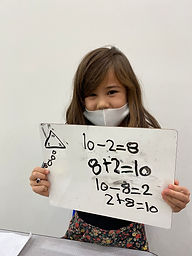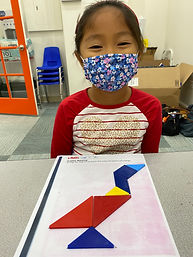## Ms. Brianne

### Target 1​

###### Lesson Type:

New

Number Operation

:

Operational Relationships

Use properties of operations as strategies for adding and subtracting.

###### 1:

Identify the Commutative Property of Addition.

###### 2:

Understand that changing the order of addends, does not change the sum.

###### 3:

Understand the inverse relationship between addition and subtraction.

1st

###### Vocabulary:

Activities:

Students

• used 3 given numbers to create 4 different equations using the same numbers
• used dice to generate numbers to create their own fact families with two addition and two subtraction equations### Home Exploration

###### Guiding Questions:## Absent Students:

### Target 2

:

###### 2:

Set the foundation for later exploration of multiplication (i.e. 2+2+2+2 =? How many 2s did you add? When you add 4 number 2s together, what do you get?)

###### 3:

Use addition to find the total number of objects in an array.

2nd

###### Vocabulary:

Row, Column, Repeat, Add, Array, Multiply, Times

Activities:

Students

• used cubes to build an array to match a multiplication equation
• used repeated addition to represent and solve their multiplication equation### Home Exploration

###### Guiding Questions:### Target 3

:

###### 1:

Solve simple tangram puzzles by recognize which spaces in the puzzle the given seven shapes can and cannot fit.

2nd

###### Vocabulary:

Tangrams, Flip, Slide, Rotate

Activities:

Students

• used tangrams to complete a puzzle using all 7 pieces
• were given the silhouette of an image and had to figure out how all the pieces fit into the silhouette### Home Exploration